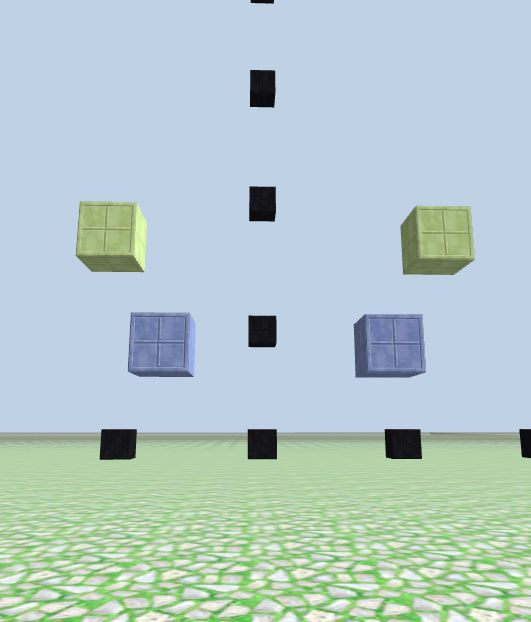# Materials Object

### `materials` is an object in CodeCraft

In earlier examples we had the following code in every program that creates blocks:

``````let game = new Game();
let materials = game.materials;
console.log(materials);
``````

As you have seen many times, the console result of the printed `materials` looks like this:

`{'box_black': 1, 'box_blue': 2,...,'tnt': 88}`

`materials` is simply an object of 88 key/value pairs.

Every key in `materials` is the name of the block material, which is a string. Its value is an integer in the range of 1 to 88, representing 88 different block materials.

### Two ways to build a diamond block

Previously we use an integer value to indicate a material when we build a block. Since `materials` is an object, we can also use `obj[key]` to access the key's value. For example: `materials['diamond']` points to integer `19`, see it in CodeCraft:

``````// build a diamond block using integer 19 indicating the material 'diamond'

game.setBlock(new Position(0, 2, -10), 19);

// build a diamond block on top of the previous one, using materials['diamond'] to access 'diamond' material

game.setBlock(new Position(0, 4, -10, materials['diamond']);
``````

From the above example you can see, using `materials['diamond']` or integer `19` will actually do the same thing: accessing the material `'diamond'`. But using the key name makes it easier to understand what material is being used to build the block. When new materials are added, the integer values may change, but the names will not.

### New function to build a block

Previously we always use integer indicating block material, such as in function `block_m(x,y,z,m)` to build a block:

``````// block_m(x, y, z, m), m is material number
function block_m(x,y,z,m) {
game.setBlock(new Position(x,y,z), m);
}
``````

But sometimes it's more convenient to just use the block's string name directly.

Similar to `block_m(x, y, z, m)`, let's define a new function called `block(x, y, z, material)`, which accepts a string name as the material input.

``````// block(x,y,z, material), material is a string such as 'brick'
function block(x,y,z,material) {
game.setBlock(new Position(x,y,z), materials[material]);
}

// column(x, z, h, material), matching with block()
function column(x,z,h,material) {
for (let j=1; j<h+1; j++) {
block(x,j,z,material);
}
}
``````

Demo the two `block` functions:

``````// blue blocks:
block_m(-2, 3, -10, 2);               # at left
block(2, 3, -10, 'box_blue');         # at right

// lime blocks:
block_m(-3, 5, -10, 8);               # at left
block(3, 5, -10, 'box_lime');         # at right
``````Both of these functions basically do the same thing, so pick whichever you want to use according to the situation.

`block(x, y, z, material)` makes it easier for you to recognize the material while you read your code.

`block_m(x, y, z, m)` is good if you want to iterate over the numbers that represent material values to change materials while building something.

From now on, we'll use `block(x, y, z, material)` and `column(x, z, h, material)` more often to make it easier to recognize the block color, and because the material number might change in the future when new materials are added.